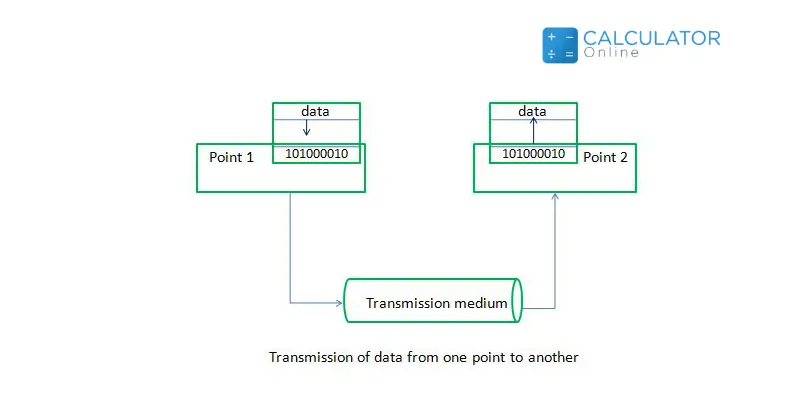Uh Oh! It seems you’re using an Ad blocker!

We always struggled to serve you with the best online calculations, thus, there's a humble request to either disable the AD blocker or go with premium plans to use the AD-Free version for calculators.

Or# Data Transfer Calculator

File Size

Transfer Speed

kilo

Table of Content

Get the Widget!

Add this calculator to your site and lets users to perform easy calculations.

Feedback

How easy was it to use our calculator? Did you face any problem, tell us!

Our Data transfer calculator helps users to calculate the time and speed to move data from one place to another, especially when transferring data from one network to another. The data transfer rate is vital to choose the bandwidth and download time of a certain Internet service provider ISP.

## How Does This Transfer Data Calculator Work?

To use this time transfer calculator, you need to follow some steps that are listed below:

Input:

• Enter the file size
• The Transfer Speed
• Submit the value of the overhead percentage
• Hit the calculate button to measure data transfer rate

Output:

The data transfer calculator generates the following results:

• The data transfer  rate (minutes, hours, day and in weeks)

## Data Transfer Speed and Duration

You can calculate the transfer speed using a network transfer speed calculator or by using the following formula:

Speed = Data Transferred / Duration.

Surprisingly, when you are going to calculate data transfer time, you need to use the same formula with a bit of rearrangements or you can use the file transfer time calculator.

Duration = Data Transferred / Speed

## Data Transfer Rate:

Data Transfer Rate (DTR) is the ratio of the total amount of digital data transferred between two points in a specific time. It can be measured manually or by using a datatransfer calculator.

DTR = Total amount of Digital data transmitted/Total time Taken
DTR= Data Transfer/Time

The formula to calculate transfer rate is:

DTR = D/T

For the more accurate measurement, you can take assistance from a data transfer bandwidth calculator.### Conversion Units of Data Transfer Rate:

The Data Transfer Rate (DTR) is measured in Megabits per second or Megabytes per second. The highest data transfer rate system in Japan is around 1414 terabits per second using only a single optical fiber cable. We need to follow the proceeding conversions to calculate data transfer rate.

Decimal Prefix Binary prefixes
1 kbit/s (kbps) = 1000 bit/s 1 kbit/s (kbps) = 1000 bit/s
1 Mbit/s (kbps) = 106 bit/s 1 Mibit/s = 220 = 10242 bit/s
1 Mbit/s (kbps) = 106 bit/s 1 Mibit/s = 220 = 10242 bit/s
1 Gbit/s (kbps) = 109 bit/s 1 Gibit/s = 230 = 10243 bit/s
1 Tbit/s (kbps) = 1012 bit/s 1 Tibit/s = 240 = 10244 bit/s

We need to calculate data transfer rate by adding the overheads of data transmission. These extra bits ensure smooth and error-free transmission of data.

How overheads or fluctuation occur in data transmission?

• Due to interference, noise, and bad connections
• Due to communication equipment
• Due to router speed

## Practical Example:

Let’s calculate the Data Transfer Rate of a 500 MB file over a 15 Mbps data connection without using a data transfer calculator.
Solution:

First:

Convert 15 Megabytes to Megabits = 15* 8= 4000 Megabits

DTR = Data Transfer/Time

DTR = 4000/15

DTR = 266.64 seconds

DTR = 4 min 26 sec

## How to Denote Units?

Name Symbol Bit Per Second Byte Per Second Bit Per Second (Formula) Byte Per Second (Formula)
Bit/Second Bit/S 1 0.125 1 1/8
Byte/Second B/s 8 1 8 1
Kilobit/Second B/s 8 1 8 1
Kibibit/Second Kibit/s 1,024 128 210 27
Kilobyte/Second kB/s 8,000 1,000 8 × 103 103
Kibibyte/Second KiB/s 8,192 1,024 213 210

## How to Transfer Data from One iPhone to Another?

The easiest way to transfer data from one iPhone to another is,

• Backup the data of your old iPhone.
• Or simply transfer your data using a QR code.

## How to Transfer Data from Android to iPhone?

To transfer the data from an android phone to iPhone, you need to use data transfer apps or simply copy the data in your laptop or PC and then transfer the data in your iPhone. If you are using the second option, try to use USB 3.0 as it has the maximum transfer speed.

## FAQs

### How do I Calculate Data Transfer Speed?

Follow the below-mentioned steps to calculate data transfer speed:

• You can get the data transfer speed by dividing the file size by measured time.

### How do I Calculate the Transfer Time of Data?

To calculate the transfer time of data, follow the steps mentioned below:

• Find the size of the data you are going to transfer.
• Determine the transfer rate of your internet.
• Divide the file size by the speed to calculate the transfer time.

### What is the Data Transfer Rate of USB 3.0?

The data transfer rate of USB 3.0 is 5 Gbps which is around 625 Mbps.

### What Factors Affect Data Transfer Speed?

The speed of data transfer can be affected by multiple factors like:

• Transfer technology used for transmission
• Location of the user
• The number of users
• The devices and peripherals
• The nature of the network(Mobile or Fixed network)

## References:

From the source of techtarget.com: DTR,Data Transfer Rate

From the source of geeksforgeeks.org: Importance of data transfer, Factors affecting data transfer rate